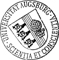Central Limit Theorems for Empirical Product Densities of Stationary Point Processes

• We prove the asymptotic normality of kernel estimators of second- and higher-order product densities (and of the pair correlation function) for spatially homogeneous (and isotropic) point processes observed on a sampling window which is assumed to expand unboundedly in all directions. We first study the asymptotic behavior of the covariances of the empirical product densities under minimal moment and weak dependence assumptions. The proof of the main results is based on the Brillinger-mixing property of the underlying point process and certain smoothness conditions on the higher-order reduced cumulant measures. Finally, the obtained limit theorems allow to construct Chi-square-goodness-of-fit tests for hypothetical product densities.

• Dokument_1.pdfAuthor: Lothar HeinrichGND, Stella Klein urn:nbn:de:bvb:384-opus4-12393 https://opus.bibliothek.uni-augsburg.de/opus4/1547 Preprints des Instituts für Mathematik der Universität Augsburg (2011-10) Preprint English Universität Augsburg 2011/07/19 Kernel-type product densities estimators; pair correlation function; Brillinger-mixing point processes; cumulant measures; large domain statistics Räumliche Statistik; Zufälliger Punktprozess; Stationärer Punktprozess; Asymptotik; Anpassungstest; Zentraler Grenzwertsatz Mathematisch-Naturwissenschaftlich-Technische Fakultät Mathematisch-Naturwissenschaftlich-Technische Fakultät / Institut für Mathematik Mathematisch-Naturwissenschaftlich-Technische Fakultät / Institut für Mathematik / Lehrstuhl für Stochastik und ihre Anwendungen 5 Naturwissenschaften und Mathematik / 51 Mathematik / 510 MathematikDeutsches Urheberrecht